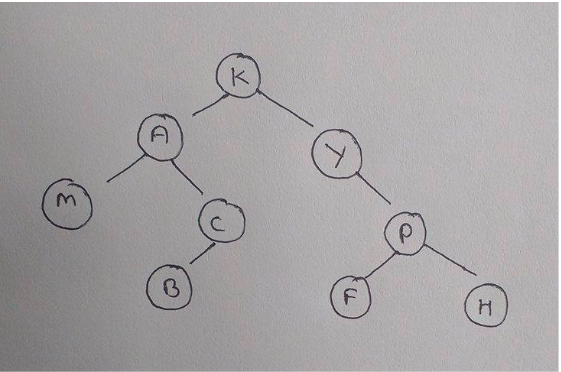GATE | Gate IT 2008 | Question 44

• Last Updated : 28 Jun, 2021

The following three are known to be the preorder, inorder and postorder sequences of a binary tree. But it is not known which is which.
MBCAFHPYK
KAMCBYPFH
MABCKYFPH
Pick the true statement from the following.

(A) I and II are preorder and inorder sequences, respectively
(B) I and III are preorder and postorder sequences, respectively
(C) II is the inorder sequence, but nothing more can be said about the other two sequences
(D) II and III are the preorder and inorder sequences, respectively

Explanation:

The approach to solve this question is to first find 2 sequences whose first and last element is same. The reason being first element in the Pre-order of any binary tree is the root and last element in the Post-order of any binary tree is the root.
Looking at the sequences given,
Pre-order   =   KAMCBYPFH
Post-order  =  MBCAFHPYK
Left-over sequence  MABCKYFPH will be in order.
Since we have all the traversals identified, let’s try to draw the binary tree if possible.I. Post order
II. Pre order
III. Inorder

This solution is contributed by Pranjul Ahuja.

Quiz of this Question

My Personal Notes arrow_drop_up